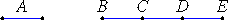# Definition 1

A unit is that by virtue of which each of the things that exist is called one.

# Definition 2

A number is a multitude composed of units.

## Guide

These 23 definitions at the beginning of Book VII are the definitions for all three books VII through IX on number theory. Some won’t be used until Books VIII or IX.

#### What are numbers?

All the numbers Euclid deals with in Books VII through IX are whole positive numbers, but there are two kinds of them. On the one hand, he uses numbers as objects of a theory, the things that are defined in Definition 2 and appear in every proposition in these books. On the other hand, he uses numbers to talk about them and to prove things about them. These are numbers in the metalanguage. They’re not multiples of the unit. Thus, there are two kinds of numbers here, the formal numbers in the object language which are multiples of the unit, and informal numbers in the metalanguage.

In the Guides, letters at the beginning of the alphabet like a, b, and c will be used for the formal numbers treated by Euclid, except u will denote the unit. Letters near the middle of the alphabet like m and n will be used for numbers in the informal metalanguage.

The barrier between the two is frequently breached as properties about the informal numbers in the metalanguage are used to prove properties about numbers in the object language. The barrier would have been stronger if Euclid had explicitly stated axioms about numbers and used them to prove properties about those numbers, but he, and his successors for over two thousand years, did not do that.

Definition 2 says that a number a is a multitude of units. If we let n be the number of units u that make up a, then we could summarize definition 2 by the equation a = nu.

Euclid never names the informal number n, but needs to refer to it frequently. For example, VII.Def.15 defines the product ab of numbers as the result when b is added as many times as there are units in a. That is, ab is the sum of n b’s where the unit u measures a n times. Thus, every formal number a = nu is shadowed by the unnamed informal number n.

Since a formal number, a, in the object language corresponds, by definition, to an unnamed informal number n in the metalanguage, it’s easy to be confused and end up, as Euclid did, proving propositions in the formal language by properties of informal numbers in the metalanguage.

#### Is the unit a number?

The numbers in definition 2 are meant to be whole positive numbers greater than 1, and Definition 1 is meant to define the unit as 1. The word “monad,” derived directly from the Greek, is sometimes used instead of “unit.”

Euclid treats the unit, 1, separately from numbers, 2, 3, and so forth. This makes his proofs awkward in some cases. Rarely Euclid does treat 1 as a number. Chrysippus (280–207), a Stoic philosopher, claimed that 1 is a number, but his pronouncement was not accepted for some time.

The numbers in definition 2 are meant to be whole positive numbers greater than 1, and definition 1 is meant to define the unit as 1. The word “monad,” derived directly from the Greek, is sometimes used instead of “unit.”

#### Representation of numbers in the ElementsThroughout these three books on number theory Euclid exhibits his formal numbers as lines. The unit is represented as one line, the line A in the diagram above. Unlike lines in the preceding books on plane geometry, this unit line A is meant to be indivisible. In the diagram, BD represents 2, and so does CE, while BE represents the number 3. Although numbers are represented as lines, Euclid never calls them lines.

It is not clear what the nature of these numbers is supposed to be, but their nature is irrelevant. Euclid could illustrate the unit as a line, a point, or a magnitude of any kind, and numbers would then be illustrated as multiples of that unit.

As mentioned above, Euclid has no postulates to elaborate the concept of number (other than the Common Notions which apparently are meant to apply to numbers as well as magnitudes of various kinds).

#### Mathematical induction

Mathematicians did not develop foundations for number theory until the late nineteenth century. The Dedekind/Peano axioms for numbers are the best known. The most important of these axioms encodes the principle of mathematical induction which states that
1. if a property of numbers holds for 1,
2. and whenever property holds for a number n then it also holds for n + 1,
3. then the property holds for all numbers.
Euclid does not use the principle of mathematical induction, but he does use a equivalent similar principle for numbers, namely, that any decreasing sequence of numbers is finite. That property is sometimes known as the descending chain condition. It’s closely related to the well-ordering principle which says that any nonempty set of numbers contains a least number.

#### The Dedekind/Peano axioms for numbers

Dedekind defined the natural numbers as a set N whose elements are called numbers, along with a specific number 1 and a successor function which associates with each number another number satisfying the following conditions

• 1 is not the successor of any number.
• No two distinct numbers have the same successor.
• The smallest subset of N that contains 1 and is closed under the successor function is N itself.
The last condition assures that the principle of mathematical induction holds on N.